Latest Banking jobs   »

# Quantitative Aptitude Quiz For SIDBI Grade A Prelims 2022- 26th December

Directions (1-5): In the following questions, two equations (I) and (II) are given. You have to solve both the equations and mark the appropriate answer.
(a) x<y
(b) x>y
(c) x≤y
(d) x≥y
(e) x=y or no relation.

Q1. I. (x+16)²=441
II. (y+22)²=961

Q2. I. x²-15x+56=0
II. y²-12y+35=0

Q3. I. x³=5832
II. y³=4096

Q4. I. (x-2)²=5x-16
II. (y-4)²=9y-56

Q5. I. 42x²-71x+30=0
II. 6y²-17y+12=0

Directions (6-10): In each of these questions, two equations (I) and (II) are given. You have to solve both the equations and give answer
(a) if x>y
(b) if x≥y
(c) if x<y
(d) if x ≤y
(e) if x = y or no relation can be established between x and y.

Q6. I. 5x² + 28x + 15 = 0
II. 3y² – 7y – 6 = 0

Q7. I. x² – 14x – 15 = 0
II. y² – 19y + 60 = 0

Q8. I. 3x + 4y = 25
II. 4x + 3y = 24

Q9. I. x² = 64
II. y² – 18y + 80 = 0

Q10. I. 7x² + 20x = –12
II. y² + 9y = –18

Directions (11-15): In each of these questions, two equations (i) and (ii) are given. You have to solve both the equations and give answer
(a) if x>y
(b) if x≥y
(c) if x<y
(d) if x ≤y
(e) if x = y or no relation can be established between x and y.

Q11. (i) 4x² + 14x – 18 = 0
(ii) y² + 12y + 35 = 0

Q12. (i) 4x² + 17x – 42 = 0
(ii) y² + 21y + 90 = 0

Q13. (i) x² – 24x + 128 = 0
(ii) y² – 34y + 288 = 0

Q14. (i) 8x² – 30x + 28 = 0
(ii) 5y² – 8y + 3 = 0

Q15. (i). 9x²-15x+6=0
(ii). 12y²+20y–8=0

Solutions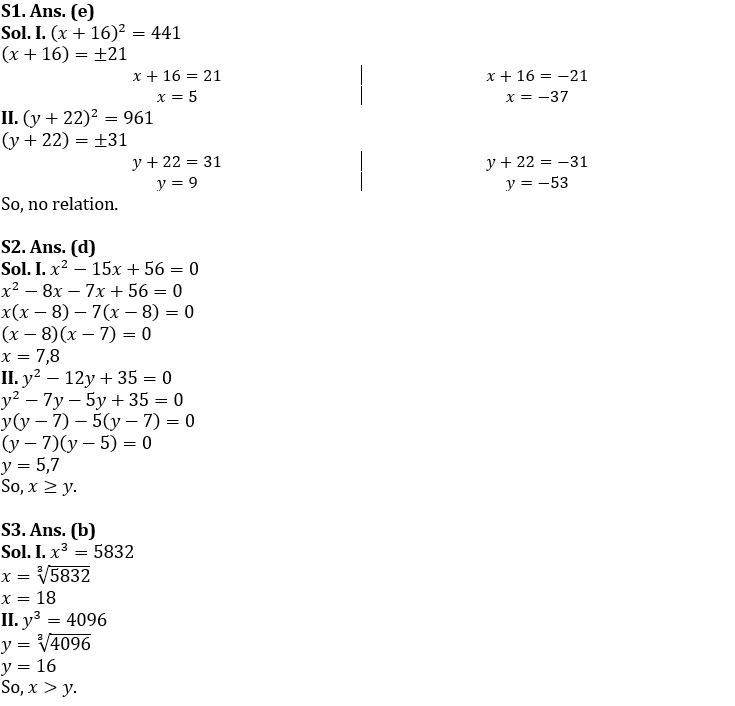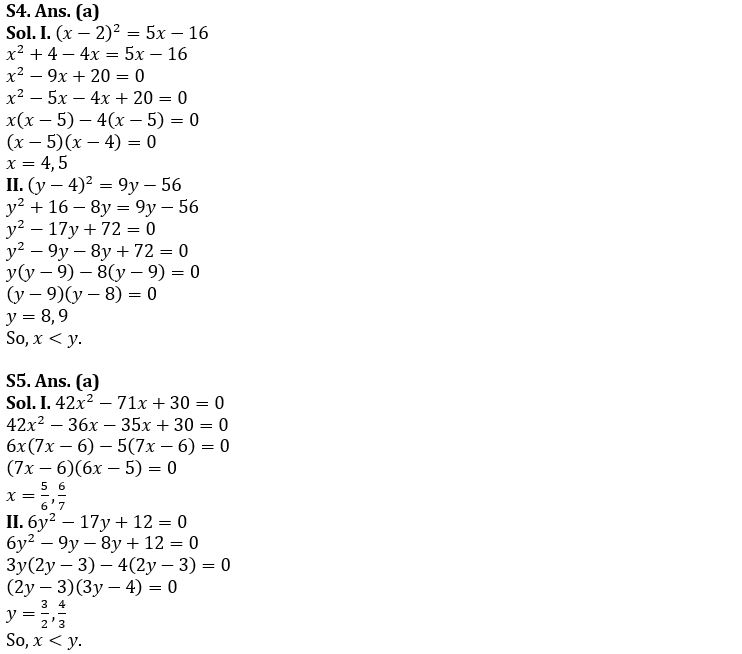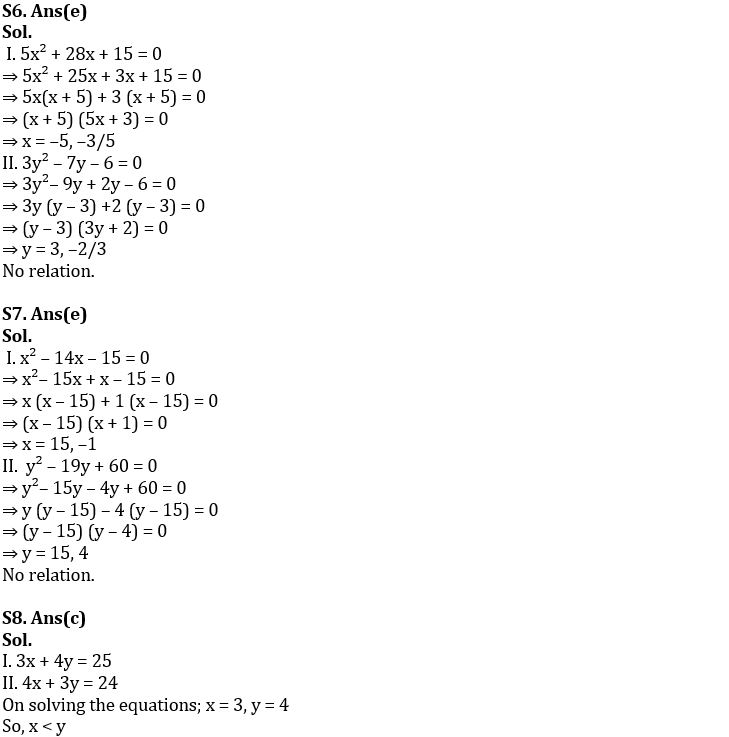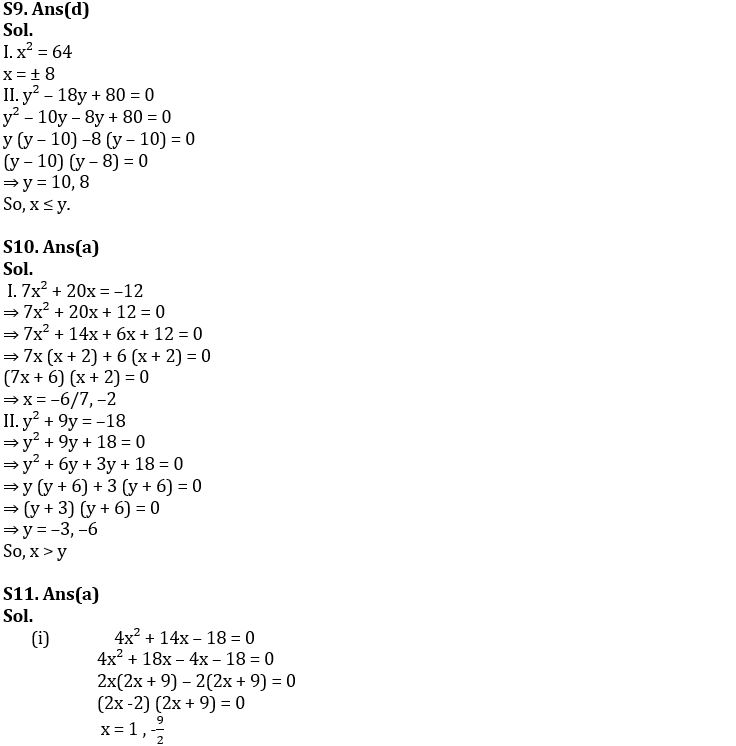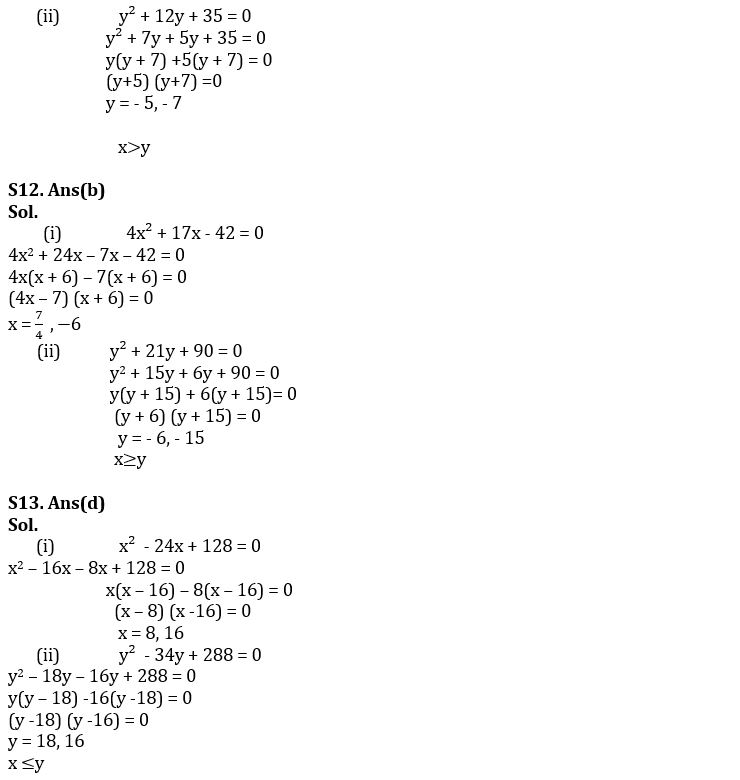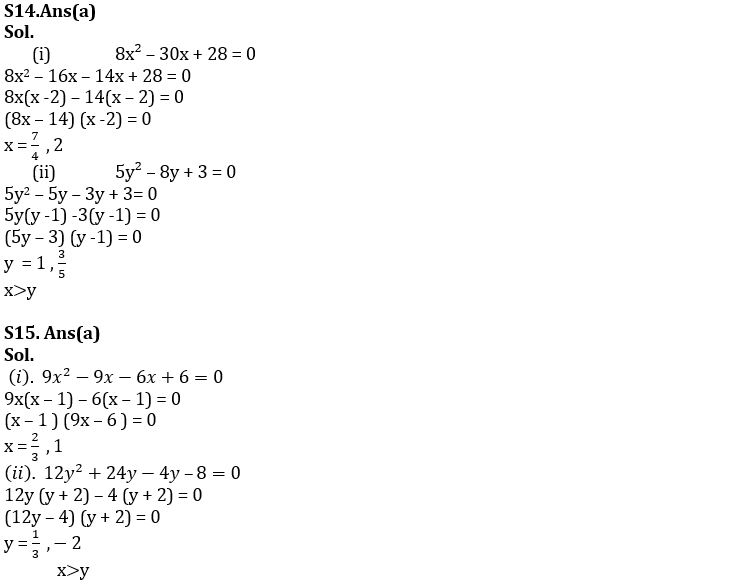#### Congratulations!Union Budget 2023-24: Free PDF